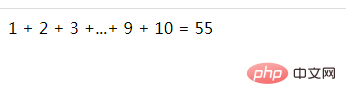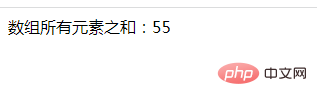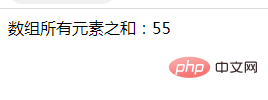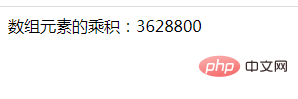# php怎么求一个数组的元素和

2种求和方式：1、遍历数组，将元素相加求和，语法“for(\$i=0;\$i<数组长度;\$i++){\$s+=\$arr[\$i];}”或“foreach(\$arr as \$v){\$s+=\$v;}”；2、直接用“array_sum(数组)”语句求和。php计算数组元素之和的方法

php计算数组元素和有多种方法，本篇文章就介绍2种求和方式，

```<?php
\$array= array(1,2,3,4,5,6,7,8,9,10);
\$sum=0;
\$len=count(\$array);//数组长度
for (\$i=0; \$i < \$len; \$i++) {
\$sum+=\$array[\$i];
}
echo '1 + 2 + 3 +...+ 9 + 10 = '. \$sum;
?>``````<?php
\$array= array(1,2,3,4,5,6,7,8,9,10);
\$sum=0;
foreach (\$array as \$value) {
\$sum+=\$value;
}
echo '数组所有元素之和：'. \$sum;
?>``````<?php
\$array= array(1,2,3,4,5,6,7,8,9,10);
echo '数组所有元素之和：'. array_sum(\$array);
?>```PHP不仅内置了一个求数组元素和的函数--array_sum()，也内置了求数组元素乘积的函数--array_product()

```<?php
\$array= array(1,2,3,4,5,6,7,8,9,10);
echo '数组元素的乘积：'. array_product(\$array);
?>```#### 相关文章推荐

• php数组如何反转• php数组怎么查询元素位置• js数组怎么转为php数组• php数组怎么实现键值不变倒序排序• php数组怎么增加键值对• php数组怎么根据key删除元素
1/1